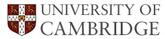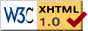# The Reconstructive Austenite-Ferrite Transformation in Low-Alloy Steels

## Abstract

A thermodynamic model has been coupled with simplified kinetic theory, so that, subject to a number of assumptions, the one- dimensional parabolic thickening constant alpha(1) for allotriomorphic ferrite growing from austenite can be estimated as a function of temperature and composition. To do this, kinetic theory for ternary Fe-C-X systems (where X represents a substantial alloying element) is extended to multicomponent alloys. Values of alpha(1) calculated assuming local equilibrium and paraequilibrium are compared. Consistent with recent calculations, the slope of the alpha(1) versus temperature plot is found to change abruptly on entry into the negligible partitioning local equilibrium regime, consistent with an increase in interfacial velocity. At very high supersaturations, the effect of the cross-terms in the diffusivity matrix appears to be small and only then can their effect be ignored. At temperatures below the Ae3', the value of alpha(1), calculated assuming local equilibrium, is less than that calculated assuming paraequilibrium. Classical nucleation theory is used to model the ferrite allotriomorphs as discs growing from prior austenite grain boundaries. It has been demonstrated that the model developed here can reproduce the C- curve behaviour typical of those parts of the time-temperature- transformation diagrams that are due to allotriomorphic ferrite, provided the paraequilibrium mode of transformation is assumed to be operative. This work therefore suggests that in multicomponent alloys, the state of true local equilibrium does not exist at the advancing interface. Some problems associated with the paraequilibrium mode of transformation during reconstructive growth are discussed.

Materials Science and Technology, Vol. 8, 1992, 421-435.

Some of the computer programs associated with this paper can be obtained from the Materials Algorithms ProjectPT Group Home Materials Algorithms